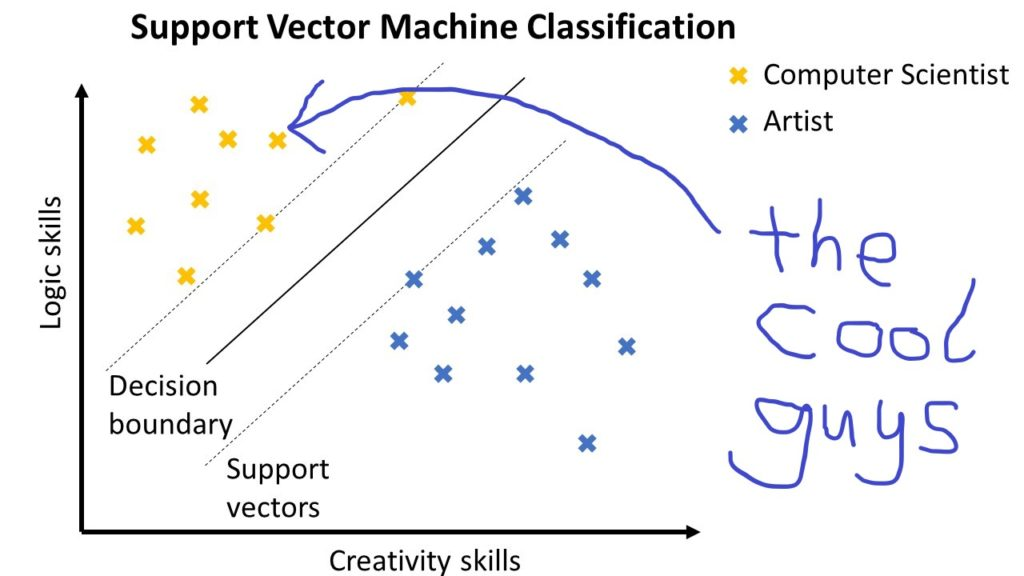[One-Liner Tutorial] Support Vector Machines Made Simple

Support Vector Machines (SVM) have gained huge popularity in recent years. The reason is their robust classification performance – even in high-dimensional spaces: Surprisingly, SVMs even work if there are more dimensions (features) than data items. This is unusual for classification algorithms because of the curse of dimensionality – with increasing dimensionality, the data becomes extremely sparse which makes it hard for algorithms to find patterns in the data set. Understanding the basic ideas of SVMs is a fundamental step to becoming a sophisticated machine learning engineer.

Before I’ll give you a cheat sheet of SVMs in Python, watch the following video that summarizes shortly how SVMs work in Python:

Here is a cheat sheet that summarizes the content of this article:

The Basics

How do classification algorithms work? They use the training data to find a decision boundary that divides data in the one class from data in the other class.

Here is an example:

Suppose, you want to build a recommendation system for aspiring university students. The figure visualizes the training data consisting of users that are classified according to their skills in two areas: logic and creativity. Some persons have high logic skills and relatively low creativity, others have high creativity and relatively low logic skills. The first group is labeled as “computer scientists” and the second group is labeled as “artists”. (I know that there are also creative computer scientists, but let’s stick with this example for a moment.)

In order to classify new users, the machine learning model must find a decision boundary that separates the computer scientists from the artists. Roughly speaking, you will check for a new user in which area they fall with respect to the decision boundary: left or right? Users that fall into the left area are classified as computer scientists, while users that fall into the right area are classified as artists.

In the two-dimensional space, the decision boundary is either a line or a (higher-order) curve. The former is called a “linear classifier”, the latter is called a “non-linear classifier”. In this section, we will only explore linear classifiers.

The figure shows three decision boundaries that are all valid separators of the data. For a standard classifier, it is impossible to quantify which of the given decision boundaries is better – they all lead to perfect accuracy when classifying the training data.

But what is the best decision boundary?

Support vector machines provide a unique and beautiful answer to this question. Arguably, the best decision boundary provides a maximal margin of safety. In other words, SVMs maximize the distance between the closest data points and the decision boundary. The idea is to minimize the error of new points that are close to the decision boundary.

Here is an example:

The SVM classifier finds the respective support vectors so that the zone between the different support vectors is as thick as possible. The decision boundary is the line in the middle with maximal distance to the support vectors. Because the zone between the support vectors and the decision boundary is maximized, the margin of error is expected to be maximal when classifying new data points. This idea shows high classification accuracy for many practical problems.

The Code

## Dependencies
from sklearn import svm
import numpy as np

## Data: student scores in (math, language, creativity) --> study field
X = np.array([[9, 5, 6, "computer science"],
[10, 1, 2, "computer science"],
[1, 8, 1, "literature"],
[4, 9, 3, "literature"],
[0, 1, 10, "art"],
[5, 7, 9, "art"]])

## One-liner
svm = svm.SVC().fit(X[:,:-1], X[:,-1])

## Result & puzzle
student_0 = svm.predict([[3, 3, 6]])
print(student_0)

student_1 = svm.predict([[8, 1, 1]])
print(student_1)

Guess: what is the output of this code?

The Results

The code breaks down how you can use support vector machines in Python in its most basic form. The NumPy array holds the labeled training data with one row per user and one column per feature (skill level in maths, language, and creativity). The last column is the label (the class).

Because we have three-dimensional data, the support vector machine separates the data using two-dimensional planes (the linear separator) rather than one-dimensional lines.  As you can see, it is also possible to separate three different classes rather than only two as shown in the examples above.

The one-liner itself is straightforward: you first create the model using the constructor of the svm.SVC class (SVC stands for support vector classification). Then, you call the fit function to perform the training based on your labeled training data.

In the results part of the code snippet, we simply call the predict function on new observations:

Because student_0 has skills maths=3, language=3, and creativity=6, the support vector machine predicts that the label “art” fits this student’s skills. Similarly, student_1 has skills maths=8, language=1, and creativity=1. Thus, the support vector machine predicts that the label “computer science” fits this student’s skills.

Here is the final output of the one-liner:

## Result & puzzle
student_0 = svm.predict([[3, 3, 6]])
print(student_0)
# ['art']

student_1 = svm.predict([[8, 1, 1]])
print(student_1)
## ['computer science']

Where to go from here?

This tutorial provides you the quickest and most concise way of starting out with support vector machines (SVMs). You won’t find any easier way on the whole Internet.

To go just one step further into the rabbit hole, and learn more about the nuances and details of SVMs, read this excellent article.

In order to implement SVMs in Python, you first need to have a solid Python foundation. To help you boost your Python skills from zero to intermediate level, I have written this carefully-crafted Python textbook “Coffee Break Python” that is 100% based on puzzle-based learning — which is scientifically proven to be one of the most effective learning methods: